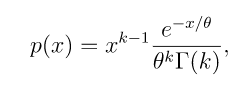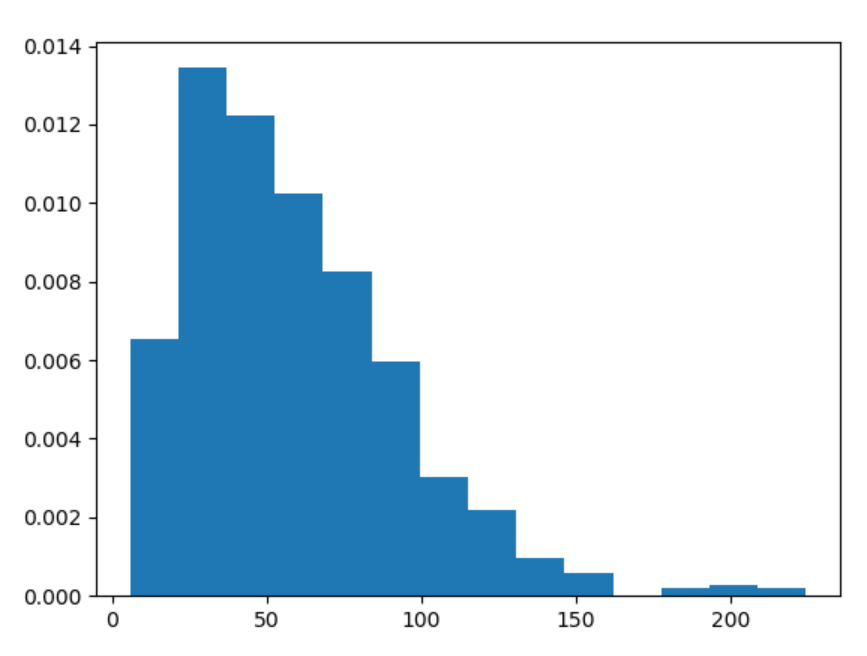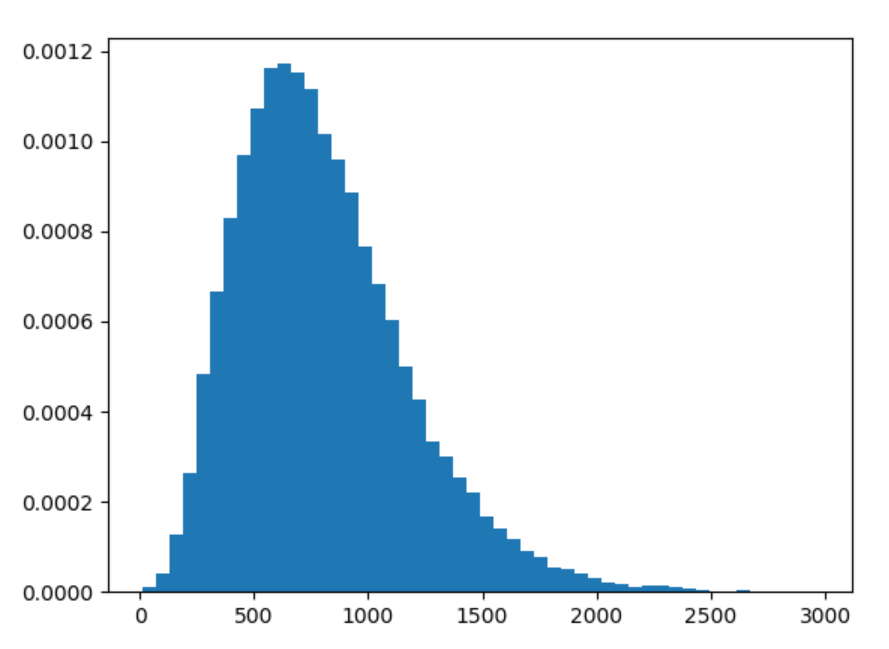Related Articles
numpy.random.gamma() in Python
• Last Updated : 15 Jul, 2020

With the help of numpy.random.gamma() method, we can get the random samples of gamma distribution and return the random samples of numpy array by using this method.gamma distribution

Syntax : numpy.random.gamma(shape, scale=1.0, size=None)

Return : Return the random samples of numpy array.

Example #1 :

In this example we can see that by using numpy.random.gamma() method, we are able to get the random samples from gamma distribution and return the random samples by using this method.

## Python3

 `# import numpy and gamma ` `import` `numpy as np ` `import` `matplotlib.pyplot as plt ` ` `  `# Using gamma() method ` `gfg ``=` `np.random.gamma(``3``, ``20``, ``1000``) ` ` `  `count, bins, ignored ``=` `plt.hist(gfg, ``14``, density ``=` `True``) ` `plt.show()`

Output :Example #2 :

## Python3

 `# import numpy and gamma ` `import` `numpy as np ` `import` `matplotlib.pyplot as plt ` ` `  `# Using gamma() method ` `gfg ``=` `np.random.gamma(``4.98``, ``12``, ``40000``) ` `gfg1 ``=` `np.random.gamma(gfg, ``13.46``, ``40000``) ` ` `  `count, bins, ignored ``=` `plt.hist(gfg1, ``50``, density ``=` `True``) ` `plt.show()`

Output :Attention geek! Strengthen your foundations with the Python Programming Foundation Course and learn the basics.

To begin with, your interview preparations Enhance your Data Structures concepts with the Python DS Course.

My Personal Notes arrow_drop_up
Recommended Articles
Page :